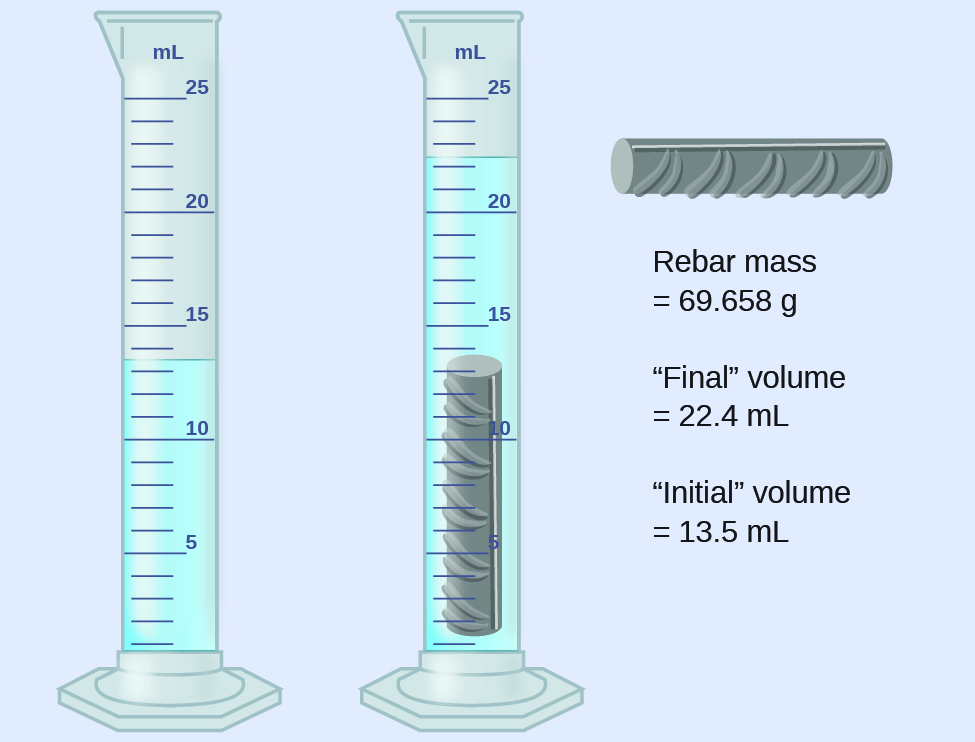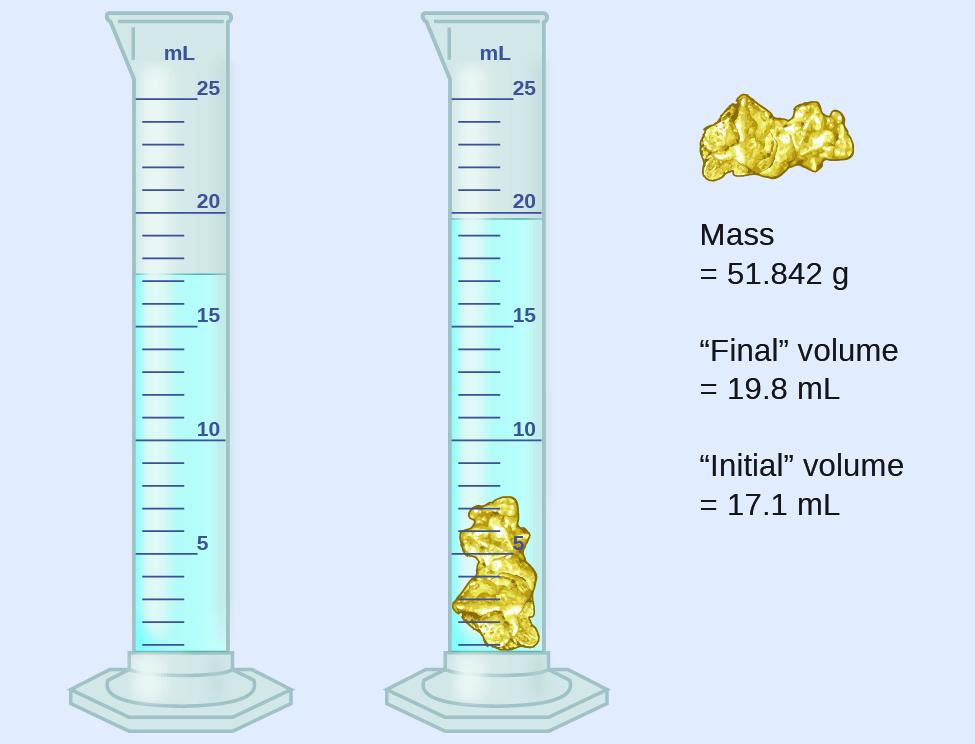# 1.5 Measurement uncertainty, accuracy, and precision  (Page 4/11)

 Page 4 / 11

## Multiplication and division with significant figures

Rule: When we multiply or divide numbers, we should round the result to the same number of digits as the number with the least number of significant figures (the least precise value in terms of multiplication and division).

(a) Multiply 0.6238 cm by 6.6 cm.

(b) Divide 421.23 g by 486 mL.

## Solution

(a) $\begin{array}{l}\begin{array}{l}\text{0.6238 cm}\phantom{\rule{0.2em}{0ex}}×\phantom{\rule{0.2em}{0ex}}6.6\phantom{\rule{0.2em}{0ex}}\text{cm}=4.11708\phantom{\rule{0.2em}{0ex}}{\text{cm}}^{2}\phantom{\rule{0.2em}{0ex}}⟶\phantom{\rule{0.2em}{0ex}}\text{result is}\phantom{\rule{0.2em}{0ex}}4.1\phantom{\rule{0.2em}{0ex}}{\text{cm}}^{2}\phantom{\rule{0.2em}{0ex}}\left(\text{round to two significant figures}\right)\hfill \\ \text{four significant figures}\phantom{\rule{0.2em}{0ex}}×\phantom{\rule{0.2em}{0ex}}\text{two significant figures}\phantom{\rule{0.2em}{0ex}}⟶\phantom{\rule{0.2em}{0ex}}\text{two significant figures answer}\hfill \end{array}\hfill \end{array}$

(b) $\begin{array}{l}\frac{\text{421.23 g}}{\text{486 mL}}\phantom{\rule{0.2em}{0ex}}=\text{0.86728... g/mL}\phantom{\rule{0.2em}{0ex}}⟶\phantom{\rule{0.2em}{0ex}}\text{result is 0.867 g/mL}\phantom{\rule{0.2em}{0ex}}\left(\text{round to three significant figures}\right)\\ \frac{\text{five significant figures}}{\text{three significant figures}}\phantom{\rule{0.2em}{0ex}}⟶\phantom{\rule{0.2em}{0ex}}\text{three significant figures answer}\end{array}$

(a) Multiply 2.334 cm and 0.320 cm.

(b) Divide 55.8752 m by 56.53 s.

(a) 0.747 cm 2 (b) 0.9884 m/s

In the midst of all these technicalities, it is important to keep in mind the reason why we use significant figures and rounding rules—to correctly represent the certainty of the values we report and to ensure that a calculated result is not represented as being more certain than the least certain value used in the calculation.

## Calculation with significant figures

One common bathtub is 13.44 dm long, 5.920 dm wide, and 2.54 dm deep. Assume that the tub is rectangular and calculate its approximate volume in liters.

## Solution

$\begin{array}{lll}V\hfill & =\hfill & l\phantom{\rule{0.2em}{0ex}}×\phantom{\rule{0.2em}{0ex}}w\phantom{\rule{0.2em}{0ex}}×\phantom{\rule{0.2em}{0ex}}d\hfill \\ & =\hfill & \text{13.44 dm}\phantom{\rule{0.2em}{0ex}}×\phantom{\rule{0.2em}{0ex}}\text{5.920 dm}\phantom{\rule{0.2em}{0ex}}×\phantom{\rule{0.2em}{0ex}}\text{2.54 dm}\hfill \\ & =\hfill & \text{202.09459}...\phantom{\rule{0.2em}{0ex}}{\text{dm}}^{3}\left(\text{value from calculator}\right)\hfill \\ & =\hfill & {\text{202 dm}}^{3}\text{, or 202 L}\phantom{\rule{0.2em}{0ex}}\left(\text{answer rounded to three significant figures}\right)\hfill \end{array}$

What is the density of a liquid with a mass of 31.1415 g and a volume of 30.13 cm 3 ?

1.034 g/mL

## Experimental determination of density using water displacement

A piece of rebar is weighed and then submerged in a graduated cylinder partially filled with water, with results as shown.(a) Use these values to determine the density of this piece of rebar.

(b) Rebar is mostly iron. Does your result in (a) support this statement? How?

## Solution

The volume of the piece of rebar is equal to the volume of the water displaced:

$\text{volume}=\text{22.4 mL}-\text{13.5 mL}=\text{8.9 mL}={\text{8.9 cm}}^{3}$

(rounded to the nearest 0.1 mL, per the rule for addition and subtraction)

The density is the mass-to-volume ratio:

$\text{density}=\phantom{\rule{0.2em}{0ex}}\frac{\text{mass}}{\text{volume}}\phantom{\rule{0.2em}{0ex}}=\phantom{\rule{0.2em}{0ex}}\frac{\text{69.658 g}}{{\text{8.9 cm}}^{3}}={\text{7.8 g/cm}}^{3}$

(rounded to two significant figures, per the rule for multiplication and division)

From [link] , the density of iron is 7.9 g/cm 3 , very close to that of rebar, which lends some support to the fact that rebar is mostly iron.

An irregularly shaped piece of a shiny yellowish material is weighed and then submerged in a graduated cylinder, with results as shown.(a) Use these values to determine the density of this material.

(b) Do you have any reasonable guesses as to the identity of this material? Explain your reasoning.

(a) 19 g/cm 3 ; (b) It is likely gold; the right appearance for gold and very close to the density given for gold in [link] .

## Accuracy and precision

Scientists typically make repeated measurements of a quantity to ensure the quality of their findings and to know both the precision    and the accuracy    of their results. Measurements are said to be precise if they yield very similar results when repeated in the same manner. A measurement is considered accurate if it yields a result that is very close to the true or accepted value. Precise values agree with each other; accurate values agree with a true value. These characterizations can be extended to other contexts, such as the results of an archery competition ( [link] ).

what is Fibonacci sequence?
what is hydrocarbons compound?
what is the 3d-orbital of Ti³+
What is Lewis acids
Lewis acid is any substance, such as the H+ ion, that can accept a pair of nonbonding electrons. In other words, a Lewis acid is an electron-pair acceptor.
betuel
Thanks
Yabsra
🤜🤛
betuel
🤜🤛
betuel
describe the way of seperation of water and kerosene
Kerosene is a hydrocarbon and non-polar. Water is a polar molecule. So a mixture of both liquids is immicible and by adding them to a separation funnel, you can open the tap flowing the less dense liquid in a container. You can read on bond polarity and separation techniques on Google.
Abdelkarim
kerosene will never with water cos its a immiscible liquid
SUNDAY
what is Chemistry
Chemistry is a branch of natural light science
Yabsra
10 sentences discussing factors affecting solubility
why is chemistry a science subject
10 sentences discussing factors affecting solubility
Sara
How to name carbonique Atom
how many period do we have in the period table
187
Ukwumonu
how do i do ionic equations
what is the formula for alkanes
Amantle
CnH2n+2 is the alkane formula.
Walter
whenever you get off your fat arse
then you can start to do some real work
hmm
gabson
How much sodium hydroxide must be dissolved in 100mL of water to prepare a 3.95molL^_1
Cindy
what is vast array
what is Nanoscience
benedict
from health care to manufacturing. Australian academy of science
what is the compound
Yaasmiin
what is Chemistry
Papie
What is array
Yabsra
what will be the total moles of all the molecule present when the different quantities of following gases are mixed together at step 4g of CH4, 22.4 dm3 of oxygen, 11.2dm3 of carbon dioxide and 3.02×10^23 molecules of ammonia.
0.5 moles of methane and 0.5 mole of sulfur dioxide are mixed together what will be the mass of mixture. a.20g b.40g c.50g d.55g e.60g
Ravina
thnx
Soni
"the halogens are all oxidizing agents" what is the reason for this observation
they are halogens....that is why numbnut 😁
This is not the place to talk down or be rude. Anonymous User keep your comments to yourself if you can't be more respectful.
DionneByByBy Brooke DelaneyBy OpenStaxBy Brooke DelaneyBy Madison ChristianBy Robert MurphyBy Yasser IbrahimBy Bonnie HurstBy John GabrieliBy Joli JuliannaBy Dewey Compton